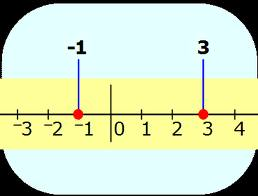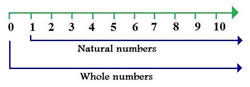# Difference between Integer and Whole Number

##### Key Difference: An integer refers to a whole number meaning that it is not in the form of a fraction. Integers comprise of whole numbers as well as their opposites.  Whole numbers are natural numbers including zero.Integers can be described as numbers that do not include any fractional or decimal component. Integers also include negative numbers. It is important to mention that 0 is also included in the list of integers. Zero is considered to be neutral which means that it is neither negative nor positive. If a number is written as a decimal or a fraction: for example, –8.00 and 12/2, both would still be referred to as integers, because they are equal to –8 and 6, respectively. Number theory is a branch of pure mathematics that is devoted primarily to the study of integers. Integers are widely used in solving problems.Whole numbers are not very different from integers. The main difference that lies between the two is that integers contain the negatives of the natural numbers, whereas whole number contains only zero and positive integers. Whole numbers are also known as counting numbers. Whole numbers are natural numbers including zero.

W = {0,1,2,3,4,5,..............}

W = 0 + N.

In order to completely understand the arithmetic of whole numbers one needs to be clear with the basic understanding of our numeral system, which is known as the Hindu-Arabic numeral system.

Comparison between Integer and Whole Number:

 Integer Whole Number Definition An integer refers to a whole number meaning that it is not in a form of a fraction. Integers comprise of whole numbers as well as their opposites. Whole numbers are natural numbers including zero. Types Positive integers - they are also know as non-negative integers.  (3,5,90) Negative integers - they are opposite of positive integers and have a prefixed sign of ‘-’. (-3, -5, -90) Positive whole numbers -  (4,6,9,10) Zero - 0 Origin of word From Latin integer (meaning literally "untouched," hence "whole": the word entire comes from the same origin, but via French. Probably from Middle English hole, unharmed,